## Solution to 1986 Problem 9

 The relevant formula is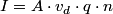\begin{align*}I = A \cdot v_d \cdot q \cdot n\end{align*}where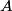$A$ is the cross-sectional area of the wire,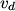$v_d$ is the drift velocity of the charge carriers,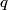$q$ is the charge of the charge carriers, and$n$ is the number density of the charger carriers. Thus,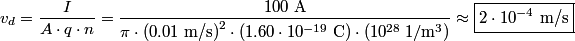\begin{align*}v_d = \frac{I}{A \cdot q \cdot n} = \frac{100 \mbox{ A}}{\pi \cdot \left(0.01 \mbox{ m/s}\right)^2 \cdot \left(... Hence, answer (D) is correct.